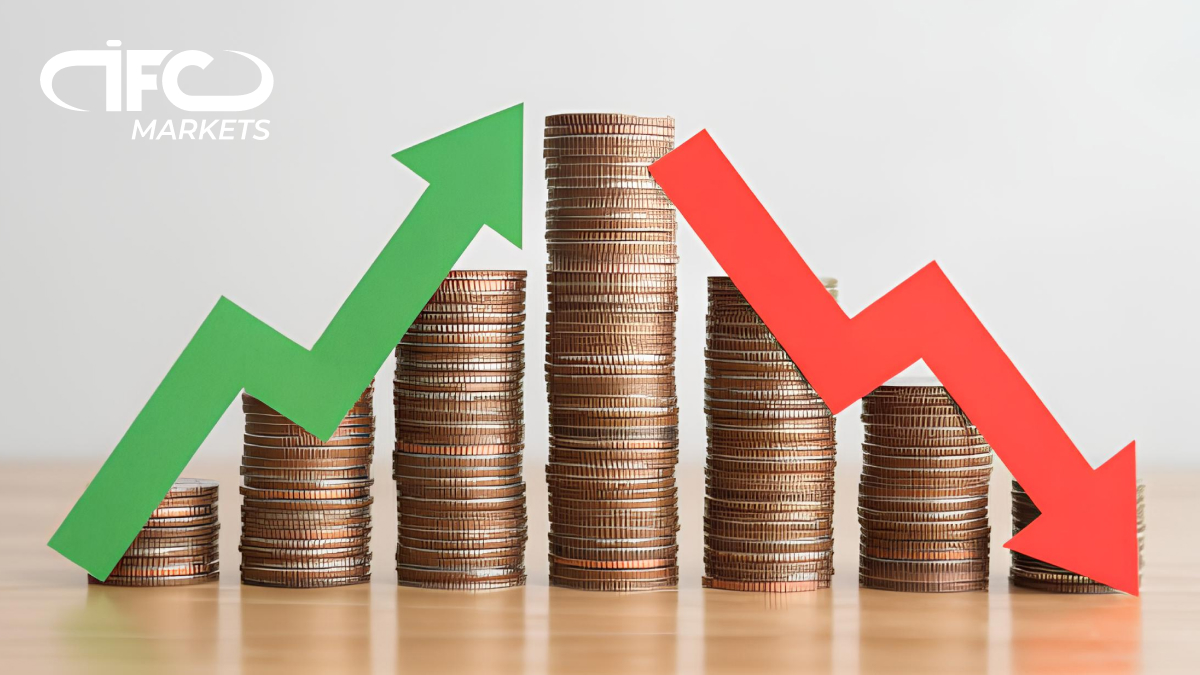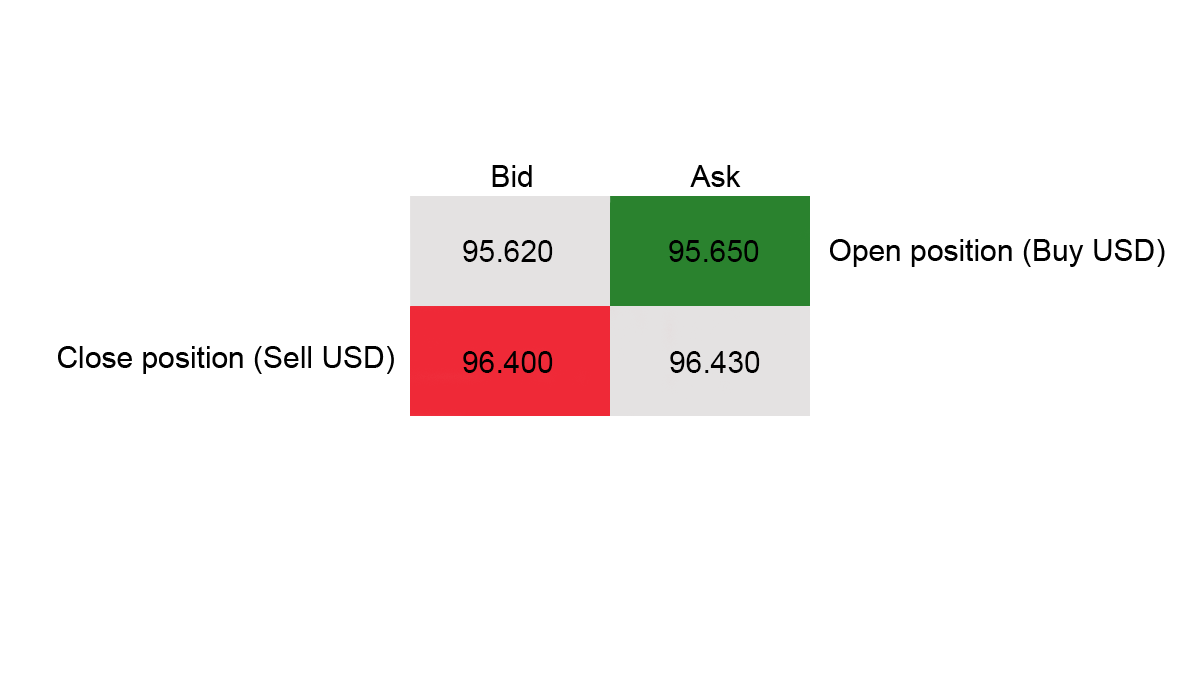How to Calculate Profit and Loss | Profit and Loss Calculation | IFCM Hong Kong
EN

# How to Calculate Profit and Loss in Trading## Profit and Loss Calculation

Each trading operation results in either profit or loss the calculation of which is performed automatically in the trading platform server. However, it is useful to know how this calculation is formulated. There are 3 important things to consider during the calculation: the volume of the opened position, the asset quotation and the direction of the position (Buy/Sell).

## How to Calculate Profit and Loss

Let us suppose the rate of USD/JPY is 95.620/95.650 and you have decided to buy 200 000 USD. Your trade is executed at the Ask price 95.650.

200 000 USD * 95.650 = 19 130 000 JPY

So, you bought 200 000 USD and sold 19 130 000 JPY.

Let us suppose that the rate of the USD/JPY changes to 96.400/96.430 and you decide to sell 200 000 USD back. The operation is performed at the Bid price 96.400.

200 000 USD * 96.400 = 19 280 000 JPYThe differential between the initial Ask and the last Bid prices is 75 pips (750 in the terminal). Thus, you bought 200 000 USD for 19 130 000 JPY and sold 200 000 USD back for 19 280 000 JPY.

The difference is your profit: 19 280 000 – 19 130 000= 150 000 JPY.

Afterwards, you can calculate this amount in USD.

150 000 JPY/96.400 = 1556.01 USD

There is also another calculation method. 1 pip value in USD/JPY is equal to 0.01, thus there is 2 000 JPY in 200 000 volume (200 000 * 0.01 = 2 000 JPY). Since the difference between the initial Ask and the last Bid price is 75 pips, we do the following: 75 * 2 000 = 150 000 JPY or 1556.01 USD.

You may also use our

Confirm the theory on practice
Once opened Demo you will be supplied with educational materials and online support